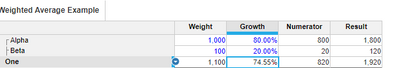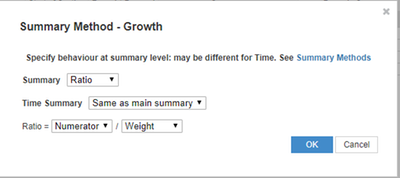# Weighted Average in Summary of a line itemHi - I have two line items, one specifying the weight and the other, say growth. For easy calculations, let's presume that the Weights and the Growth values are manual input. Growth is a manual input only at lower level - say a different line item is created for manual input. Now, when someone views the Growth at the upper level in the hierarchy, I need the system to calculate the weighted average instead of the normal average summary method.

Example: Level 1 is the higher level. Level 2 is the lower level. Under a level 1 list member, there are two 'Level 2' list members. And the weights for these two 'Level 2' list members are 1000 and 100 respectively (say). Growth is given for these two list members as 80% and 20%. If I keep the average summary for the Growth line item, it is seen at 50% at level 1 hierarchy. But what is expected is: (1000*80+100*20) / (1000+100) = 74.54%. In a similar fashion, if we have multiple levels in the hierarchy, the upper level has to take the weights at the upper level and the calculated growth at the upper level and then do the weighted average.

Thanks,

Regards - Guru

Tagged:

•There is no direct way to get the weighted averages. But if you have the logic (which in this case you seem to have one ) you can make it work thru Ratio Summary Levels

I have quickly created something, see if this helpsSummary Level of Growth Line item which is based on the Calculations done.•Hi Guru,

I haven't tried to mimick it. But try the following to ensure I understand you correctly:

Add a line item: "weighted growth" => at the lower level you specify.

Doesn't the aggregation do the work for you then? Or by adjusting those summary settings to Formula?

Feel free to share some screenshots to make it more visual, it's pretty abstract in this way.

All the best,

Maarten.

•Misbah's recommendation did work for me. Thanks.
•This works if we have few lines only. In case its a Costing model implementation, it actually gets difficult to do for most of the lines. Considering space and time.